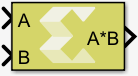# Matrix Multiply - 2022.1 English

## Vitis Model Composer User Guide (UG1483)

Document ID
UG1483
Release Date
2022-05-26
Version
2022.1 English

Compute matrix product of two input signals. The first operand is the top input on the block.

## Library

Math Functions / Matrices and Linear Algebra## Description

The Matrix Multiply block has two input ports and one output port. The output signal is the matrix product of the input signals where the first operand corresponds to the top input.

## Data Type Support

The data type of the input signals can be any floating-point, fixed-point, integer, or Boolean. The input signals can be real or complex. The input signals can be scalar, vector, or matrix, but they do need to be such that mathematically, the matrix product is defined. The table below shows valid combinations. Combinations that do not match any row in the table result in an error.

Table 1. Data Type Combinations
Dimensions of First Operand Dimensions of Second Operand Dimensions of Matrix Product Conditions
K x L L x M K x M K >= 1, L >= 1, M >= 1
K x L L K K >= 1, L > 1
K x 1 1 K x 1 K >= 1
K 1 K K >= 1
K 1 x M K x M K >= 1, M >= 1

The output data type is determined according to the following rules, in the order listed. T1 is a variable representing the type of the first operand; T2 is a variable representing the type of the second operand. These rules were chosen for maximum alignment with Vitis HLS, which may not correspond to the output data type computed via the internal rule of the Simulink® Matrix Product block.

Table 2. Output Data Type
Data Type of First Operand Data Type of Second Operand Data Type of Matrix Product
T1: floating-point T2 The widest floating-point type between T1 and T2 if T2 is a floating-point type; otherwise T1
T1 T2: floating-point The widest floating-point type between T1 and T2 if T1 is a floating-point type; otherwise T2
fixed-point fixed-point The smallest fixed-point type capable of representing the product without loss of precision
fixed-point integer The smallest fixed-point type capable of representing the product without loss of precision
integer fixed-point The smallest fixed-point type capable of representing the product without loss of precision
T1: integer T2: integer Let W1 be the bit width of T1 and W2 be the bit width of T2. The product is the integer type with bit width max (W1,W2) and it is signed if either T1 or T2 are signed.
boolean T2 T2
T1 boolean T1

## Parameters

The Matrix Multiply block has no parameters to set.